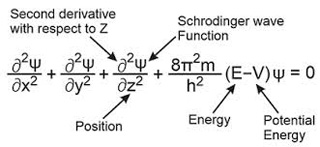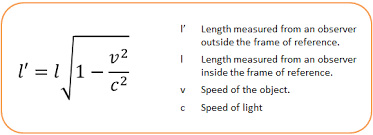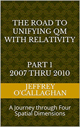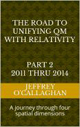# The Physicality of Schrödinger’s wave function.

There can be no doubt the probabilistic interpretation of SchrÃ¶dinger’s wave function predicts with amazing precision the results of every experiment involving the quantum world that has ever been devised to test it.

However, defining our world in this manner presents a problem because that equation defines all its possible configurations that it can have before one makes an observation.  In quantum mechanical jargon when an "observation" is made the wave function which initially is in a superposition of all possible eigenstates collapses and appears to reduce to a single eigenstate due to interaction with the external world.But the question then become what happened to all of the other possibilities.  Because according to quantum mechanical interpretation of the wave equation they are just a real as the one which we observe.

Many physicists have attempted to provide an explanation as what happens to these other possibilities because quantum mechanics would not be valid without them.

One of the most bazaar, in my option is the many-worlds interpretation because it that asserts the objective reality of the wave function and denies the actuality of its collapse.  Briefly it implies that all possible alternate histories and futures are real, each representing an actual "world" (or "universe") and that when an observation is made. In layman’s terms, the hypothesis states there is a very large; perhaps infinite number of parallel universes, and everything that could possibly have happened in our past, but did not, has occurred in the past of some other universe or universes.

However, we may be able to find a much simpler and less bazaar explanation of what actually happens to the wave function when an observation is made if we interrupt it terms of the physicality which can associated it with instead of its abstract mathematical properties. One

thing that I am pretty sure we can all agree on is that an energy associated with transverse wave define by it consist of alternating spatial displacements that extend outward from is source.

We also know that Einstein define gravitational energy in terms a positive curvature or distortion in the geometry of a universe consisting of space-time however, if we are to be consistent with Einstein definition of gravity, we must define traverse properties of the wave function in terms of the physicality of a transverse wave in the geometry of the universe. Yet one can only define the transverse properties of a wave with respect to the spatial not time properties of our universe. Therefore, to integrate it with Einstein’s theories one must be able to derive the energy associated with it in terms of the spatial properties of a transverse wave in the geometry of our universe.

Einstein gave us the ability to do this when he used the equation E=mc^2 and the constant velocity of light to define the geometric properties of space-time because it provided a method of converting a unit of time he associated with energy to unit of space.   Additionally, because the velocity of light is constant, he also defined a one to one quantitative correspondence between the both the relativistic and physical properties of a space-time universe and one made up of only four *spatial* dimensions.

However doing so would allow one to derive the probabilistic interpretation of SchrÃ¶dinger’s wave equation and quantum properties of a particle in terms of the laws of classical wave mechanics.

For example, the article “Why is energy/mass quantized?” Oct 4, 2007 showed one can derive the quantization of a wave on a "surface" of a three-dimensional space manifold with respect to fourth spatial dimension by extrapolating our understanding of a resonant structure and perception of a wave in a three-dimensional environment.

Briefly it showed the four conditions required for resonance to occur in a three-dimensional environment, an object, or substance with a natural frequency, a forcing function at the same frequency as the natural frequency, the lack of a damping frequency and the ability for the substance to oscillate spatial would occur in one consisting of four spatial dimensions.

The existence of four *spatial* dimensions would give an electromagnetic wave the ability to oscillate spatially on a "surface" between a third and fourth *spatial* dimensions thereby fulfilling one of the requirements for classical resonance to occur.

These oscillations would be caused by an event such as the decay of a subatomic particle or the shifting of an electron in an atomic orbital. This would force the "surface" of a three-dimensional space manifold to oscillate with the frequency associated with the energy of that event.

The oscillations caused by such an event would serve as forcing function allowing a resonant system or "structure" to be established space.

Therefore, these oscillations in a "surface" of a three-dimensional space manifold would meet the requirements mentioned above for the formation of a resonant system or "structure" in four-dimensional space if one extrapolated them to that environment.

In our three-dimensional environment the energy of a resonant system can only take on the discrete or quantized values associated with its fundamental or a harmonic of its fundamental frequency.

Hence, these resonant systems in four *spatial* dimensions would be responsible for the discrete quantized energy associated with the quantum mechanical properties of a photon or an electromagnetic field.

Yet one can also define its boundary conditions of its resonate structure in the terms of our perceptions of a three-dimensional environment.

For example, in our three-dimensional world, a point on the two-dimensional surface of paper is confined to that surface. However, that surface can oscillate up or down with respect to three-dimensional space.

Similarly, an object occupying a volume of three-dimensional space would be confined to it however, it could, similar to the surface of the paper oscillate “up” or “down” with respect to a fourth *spatial* dimension.

The confinement of the “upward” and “downward” oscillations of an energy wave with respect to a fourth *spatial* dimension by an observation is what defines the spatial boundaries associated with a particle in the article “Why is energy/mass quantized?" Oct 4, 2007.

Additionally, one of the most advantageous results of viewing the relativistic properties of Einstein’s theories in terms of their spatial instead of their time components is that it allows for the integration of one of most perplexing aspects of quantum mechanics; that of how and why a particles position when observed is based on probabilities.

The physics of wave mechanics tell us that due to their continuous properties the energy waves the article "Why is energy/mass quantized?" Oct. 4, 2007 associated with a quantum system would be distributed throughout the entire "surface" a three-dimensional space manifold with respect to a fourth *spatial* dimension.

For example, the energy of a vibrating or oscillating ball on a rubber diaphragm would be disturbed over its entire surface while the magnitude of those vibrations would decrease as one move away from the focal point of the oscillations.

Similarly, if the assumption that quantum properties of energy are a result of vibrations or oscillations in a "surface" of three-dimensional space is correct those oscillations would be distributed over the entire "surface" three-dimensional space while the magnitude of those vibrations would be greatest at the focal point of the oscillations and decreases as one moves away from it.(Some may question the fact that the energy wave associated with particle would be simultaneously distributed over the entire universe.  However, the relativistic properties of space time tell us the distance perceived by objects or particles in relative motion is dependent on their velocity which become zero at the speed of light.  Therefore, from the perspective of an energy wave moving at the speed of light, the distance between all points in the universe along its velocity vector is zero.  In other words, because its energy is moving at the speed of light it would distributed or simultaneous exists at every point in the universe along its velocity vector.  There can be no other conclusion if one accepts the validity of Einstein’s theories.)

As mentioned earlier the article “Why is energy/mass quantized?” shown a quantum particle is a result of a resonant structure formed on the "surface" of a three-dimensional space manifold with respect to a fourth *spatial* dimension.

Yet the science of Wave Mechanics tells us resonance would most probably occur on the surface of the rubber sheet were the magnitude of the vibrations is greatest and would diminish as one move away from that point,

Similarly, a particle would most probably be found were the magnitude of the vibrations in a "surface" of a three-dimensional space manifold is greatest and would diminish as one move away from that point.

In quantum mechanical jargon when an "observation" is made of the energy represented by the wave function which as mentioned earlier would be distributed throughout the entire universe collapses and is reduce to the single eigenstate due to interaction with the external world.

This shows how one can physically connect the probabilities associated quantum mechanics to our observable environment by redefining them in terms of the relativistic properties of Einstein’s space-time universe in terms of four *spatial* dimensions.

In other words by changing our interoperation of SchrÃ¶dinger’s wave function from a mathematical one to the physicality of a transverse wave in either four *spatial* dimensions or four dimensional space-time will allow us to ingrate the world of quantum mechanics into the space-time-universe defined by Einstein because as was shown above its probabilistic mathematical interpretation can be predicted by using them.It should be remembered that Einstein’s genius allows us to choose whether to create physical images of an unseen "reality" in either a space-time environment or one consisting of four *spatial* dimension when he defined the geometry of space-time in terms of the constant velocity of light.

Later Jeff

 The Road to Unifying QM with Relativity part 1 2007 thru 2010Ebook \$8.00Paper Back \$15.00 The Road to Unifying QM with Relativity part 2 2011 thru 2014Ebook \$8.00Paper Back \$16.00 The Road to Unifying QM with Relativity part 3 2015 thru 2020Ebook \$8.00Paper Back \$18.00